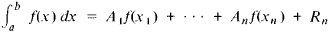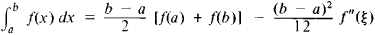formulas for approximating definite integrals by means of the values of the integrand at a finite number of points. The most common quadrature formulas have the formwhere x1x2, …, xn are the nodes of the quadrature formula, A1, A2 . . . ,An are its coefficients, and Rn is the remainder term. For example,where a ≤ ξ ≤ b (trapezoidal rule). Sometimes for mulas for mechanical, or numerical, quadrature are also called quadrature formulas.

### REFERENCE

Krylov, V. I. Priblizhennoe vychislenie integralov, 2nd ed. Moscow, 1967.
References in periodicals archive ?
In sparse grids, quadrature formulas of high order for some dimensions are combined with quadrature formulas of lower order for the remaining dimensions in a way that the interpolation error is equivalent to that of full tensor products.
They show how to use exact-difference schemes as a starting point for constructing finite-difference schemes suitable for implementation on a computer, and show that combining exact-difference schemes with modern quadrature formulas results in numerical algorithms that are highly efficient.
Indeed, by using this representation, we introduce a class of weighted interpolatory quadrature formulas in Section 3.
In order to model the triangles with curved edges in the boundary of the area more accurately, curvilinear coordinate transformation is used to map a curved triangle to a straight one; then the high order symmetrical Gaussian quadrature formulas over the triangles are adopted to evaluate the finite Fourier integration (1) more accurately; finally the 2D NUFFT  algorithm helps to make the computational complexity similar to 2D FFT.
Now if f is as above, then we have the one-point and two-points open Newton-Cotes quadrature formulas
Quadrature formulas based on rational interpolation.
Gauss-Szego quadrature formulas [20,22,39] are formulas of maximal degree for estimating integrals with respect to measures with support on the unit circle.
i,v] in Gauss-Turan quadrature formulas with multiple nodes and their generalizations can be found in [15, 18, 28, 37] (see also ).
The possibility of a "subperiodic" trigonometric quadrature formula with positive weights opens the way to construct stable product quadrature formulas [17, Chapter 2], which are exact for total-degree algebraic polynomials on domains related to circular arcs.
The Verblunsky coefficients play an important role in the construction of Szego quadrature formulas on the unit circle; see .
The quadrature formulas are interpolatory and therefore the degree of exactness is at least n.

Site: Follow: Share:
Open / Close